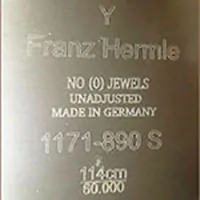# hermle-dates

July 14, 2019 8:31 am Leave your thoughts### Date Code Explanations

Hermle clock movement date codes are explained in this section. This is so you know when the movement was made and decide if the clock has lifed its life and its time for a new one. After figuring out the date code, figure out how old it is so you can justify the purchase of a new one with ease. If your clock is over 20 years old, replace it. If the clock is under 20 years old, consider fixing it instead.

Early movements prior to the year 1987 have just the stamp of the year on the back of the clock movement, 81 just means 1981.

From 1987 on, they used letter codes instead of numbers to represent the year made. Such as the year 1987 is only marked with the letter A instead, and 1988 is the letter B.

### Letter Code Definitions

This section explains the letters that sometimes come after the movement numbers on a Hermle clock movement.

These letters often can be ignored in replacing a movement with us, we offer movements by the features instead. The letters on the new movement may or may not be the same on the new one, but you need not concern yourself with this. The features of the movement would be the same, such as if you had a second bit before and the movement number did not have an S, but the new one does have an S.

• DB = Dead Beat
• CS = Small Suspension -OR- Seconds and Made in Germany
• C = Engineering Code
• D = Engineering Code
• K = Calendar -OR- Made in Germany
• S = Seconds -OR- Kit Format 132-071
• SE = Swiss Escapement
• N = Low Bridge
• H = High Bridge
 76 = 1976 77 = 1977 78 = 1978 79 = 1979 80 = 1980 81 = 1981 82 = 1982 83 = 1983 84 = 1984 85 = 1985 86 = 1986 87 = 1987 A = 1988 B = 1989 C = 1990 D = 1991 E = 1992 F = 1993 G = 1994 H = 1995 I = 1996 J = 1997 K = 1998 L = 1999 M = 2000 N = 2001 O = 2002 P = 2003 Q = 2004 R = 2005 S = 2006 T = 2007 U = 2008 V = 2009 W = 2010 X = 2011 Y = 2012 Z = 2013 AA = 2014 AB = 2015 AC = 2016 AD = 2017 AE = 2018 AF = 2019